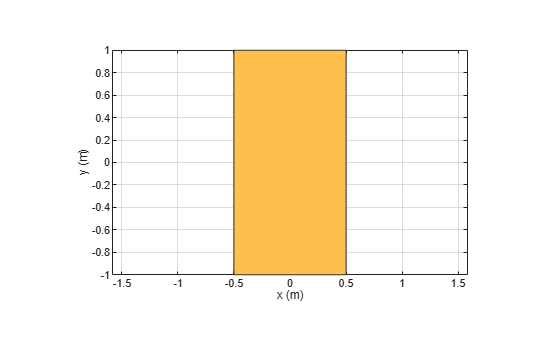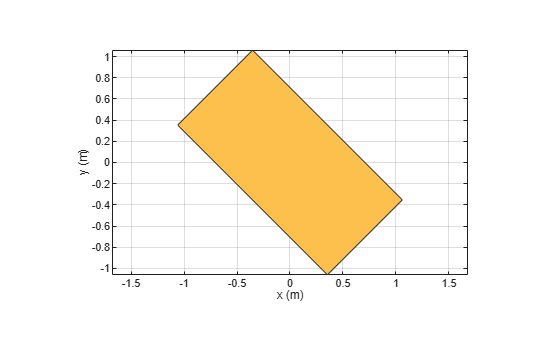# rotate

Rotate shape about axis by angle

## Syntax

``rotate(shape,angle,axisPoint1,axisPoint2)``
``c = rotate(shape,angle,axisPoint1,axisPoint2)``

## Description

example

````rotate(shape,angle,axisPoint1,axisPoint2)` rotates the shape about an axis by an angle and plots the resultant shape.```
````c = rotate(shape,angle,axisPoint1,axisPoint2)` rotates shape about an axis and an angle and returns a shape object.```

## Examples

collapse all

Create a rectangle shape.

```r = antenna.Rectangle; show(r) axis equal```Rotate the rectangle at 45 degrees about the Z-axis.

`r1 = rotate(r,45,[0 0 0],[0 0 1])`
```r1 = Rectangle with properties: Name: 'myrectangle' Center: [0 0] Length: 1 Width: 2 NumPoints: 2 ```
`show(r1)`## Input Arguments

collapse all

2-D and 3-D geometric shapes created using shape objects in Custom 2-D and 3-D Antenna catalog.

Example: `antenna.Rectangle`

Example: `shape.Polygon`

Example: `shape.Box`

Axis of rotation defined as a line joining two points, specified as two unique three-element vectors of Cartesian coordinates in meters.

Example: `[0 0 0], [0 0 1]`

Data Types: `double`

Angle of rotation, specified as a scalar in degrees.

Example: `45`

Data Types: `double`

## Output Arguments

collapse all

Resultant shape obtained after the rotation operation on the input shape, returned as a shape object.

Example: `antenna.Rectangle`

Example: `shape.Polygon`

Example: `shape.Box`

## Version History

Introduced in R2017a